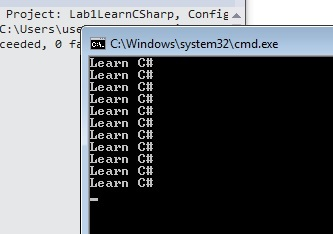# IF Conditions and FOR Loops in CSharp

##### How to Write IF Conditions and FOR Loops in C#?

One of the things in programming we always need is conditional checks. Mean if this condition is satisfied then execute some part of code or else execute something else.

So to write “IF” condition we put condition inside the round brackets ( “(“ ) and the code to be executed inside curly brackets “{“. The code which we want to execute If the condition is not satisfied is written in the “else” part.

```if (str.Length != 0)
{
// Do this
}
else
{
// or else Do this
}
```

The other thing we need in any programming logic is executing some code in a loop. Now the loop can be a finite loop or an infinite one. In order to execute code in a loop we need to use the “for” syntax as shown in the code below. “for” takes three parameters :-

• The first parameter “int i =0” initializes the variable. So this for loop will start from “0”.
• The second parameter is the condition till which this for loop should run. In this case it will run 9 times. If you can guess why I said 9 times and why not 10 times?
• The third parameter says will the variable increment or decrement.

```for (int i = 0; i < 10; i++)
{

}
```

So below is a simple C# sample code which demonstrates the use of “if” condition and “for” loop. In the below sample we are reading the input from the keyboard and then displaying that inputted value 10 times.

```string str = Console.ReadLine().ToString(); // read data from the input keyboard
if (str.Length != 0)
{
for (int i = 0; i < 10; i++)
{
Console.WriteLine(str); // display what is entered in the str variable
}
}
```

Below is how the output should look like.Views: 14900 | Post Order: 11